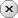### 用户注册### 用户登录### 发表随想# 用户预订类，实现预订信息的管理，包括预定编号、预订起止日期等

2020-08-13 作者：云代码会员举报

## [java]代码库

```package BookingGym.model;

import java.util.Date;

import java.util.Objects;

public class Reservation {

private int r_id_reservation;

private int r_id_client;

private String r_hall;

private Date r_begin_visits;

private Date r_end_visits;

private int r_id_tariff;

private int r_id_status;

public Reservation(){}

public Reservation(int r_id_reservation, int r_id_client, String r_hall, Date r_begin_visits, Date r_end_visits, int r_id_tariff, int r_id_status) {

this.r_id_reservation = r_id_reservation;

this.r_id_client = r_id_client;

this.r_hall = r_hall;

this.r_begin_visits = r_begin_visits;

this.r_end_visits = r_end_visits;

this.r_id_tariff = r_id_tariff;

this.r_id_status = r_id_status;

}

public int getR_id_reservation() {

return r_id_reservation;

}

public void setR_id_reservation(int r_id_reservation) {

this.r_id_reservation = r_id_reservation;

}

public int getR_id_client() {

return r_id_client;

}

public void setR_id_client(int r_id_client) {

this.r_id_client = r_id_client;

}

public String getR_hall() {

return r_hall;

}

public void setR_hall(String r_hall) {

this.r_hall = r_hall;

}

public Date getR_begin_visits() {

return r_begin_visits;

}

public void setR_begin_visits(Date r_begin_visits) {

this.r_begin_visits = r_begin_visits;

}

public Date getR_end_visits() {

return r_end_visits;

}

public void setR_end_visits(Date r_end_visits) {

this.r_end_visits = r_end_visits;

}

public int getR_id_tariff() {

return r_id_tariff;

}

public void setR_id_tariff(int r_id_tariff) {

this.r_id_tariff = r_id_tariff;

}

public int getR_id_status() {

return r_id_status;

}

public void setR_id_status(int r_id_status) {

this.r_id_status = r_id_status;

}

@Override

public int hashCode() {

int hash = 5;

hash = 89 * hash + (int) (this.r_id_reservation ^ (this.r_id_reservation >>> 32));

hash = 89 * hash + (int) (this.r_id_client ^ (this.r_id_client >>> 32));

hash = 89 * hash + Objects.hashCode(this.r_hall);

hash = 89 * hash + Objects.hashCode(this.r_begin_visits);

hash = 89 * hash + Objects.hashCode(this.r_end_visits);

hash = 89 * hash + (int) (this.r_id_tariff ^ (this.r_id_tariff >>> 32));

hash = 89 * hash + (int) (this.r_id_status ^ (this.r_id_status >>> 32));

return hash;

}

```

### 网友评论    (发表评论)### 评论须知：

• 1、评论每次加2分，每天上限为30；
• 2、请文明用语，共同创建干净的技术交流环境；
• 3、若被发现提交非法信息，评论将会被删除，并且给予扣分处理，严重者给予封号处理；
• 4、请勿发布广告信息或其他无关评论，否则将会删除评论并扣分，严重者给予封号处理。